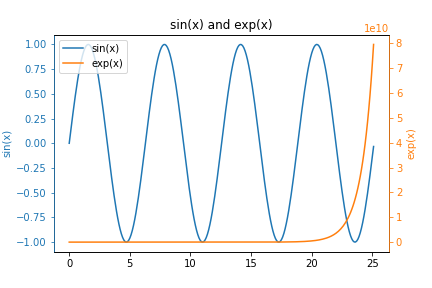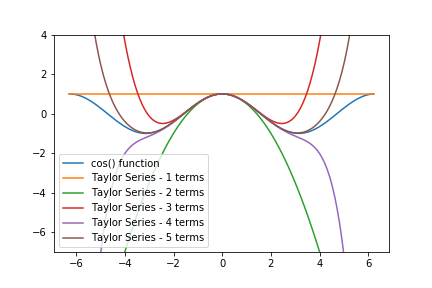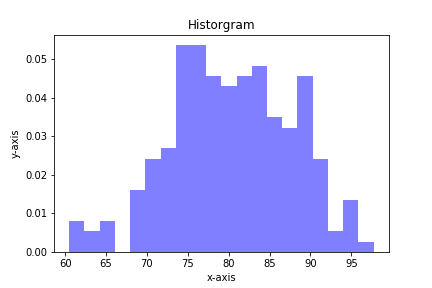## How to make y-y plots with MatplotlibIn this post, you will learn how to create y-y plots with Python and Matplotlib. y-y plots are a type of line plot where one line corresponds to one y-axis and another line on the same plot corresponds to a different y-axis. y-y plots typically have one vertical y-axis on …

## Plotting Bond Energy vs. Distance with Python and MatplotlibAtoms in solid materials like steel and aluminum are held together with chemical bonds. Atoms of solid materials are more stable when they are chemically bonded together, and it takes energy to separate atoms which are joined together with a chemical bond. The bonding energy associated with a chemical bond …

## Taylor Series in PythonIn this post, we will review how to create a Taylor Series with Python and for loops. Then we will refactor the Taylor Series into functions and compare the output of our Taylor Series functions to functions from Python's Standard Library.

A Taylor Series is an infinite series of mathematical …

## Quiver plots using Python, matplotlib and Jupyter notebooksA quiver plot is a type of 2D plot that shows vector lines as arrows. Quiver plots are useful in electrical engineering to visualize electrical potential and valuable in mechanical engineering to show stress gradients. In this post, we will build three quiver plots using Python, matplotlib, numpy, and Jupyter notebooks.

## Plotting Histograms with matplotlib and PythonHistograms are a useful type of statistics plot for engineers. A histogram is a type of bar plot that shows the frequency or number of values compared to a set of value ranges. Histogram plots can be created with Python and the plotting package matplotlib. The plt.hist() function creates …